【转载】没有博士学位，照样玩转TensorFlow深度学习

1806 9

快来加入 TensorFlowers 大家庭！

x

1、概述①神经网络的定义及如何训练神经网络
②如何使用 TensorFlow 构建基本的 1 层神经网络
③如何添加多层神经网络
④训练提示和技巧：过拟合、dropout、学习速率衰减等...
⑤如何解决深度神经网络的问题
⑥如何构建卷积网络

①Python 2 或 3（建议使用 Python 3）
②TensorFlow
③Matplotlib（Python 的可视化库）

2. 准备：安装 TensorFlow，获取示例代码

$git clone https://github.com/martin-gorner/tensorflow-mnist-tutorial 这个库包含了多个文件，而你将只在mnist_1.0_softmax.py中操作。其它文件是用于加载数据和可视化结果的解决方案或支持代码。 当你启动初始python脚本时，应当能够看到训练过程的实时可视化：$ python3 mnist_1.0_softmax.py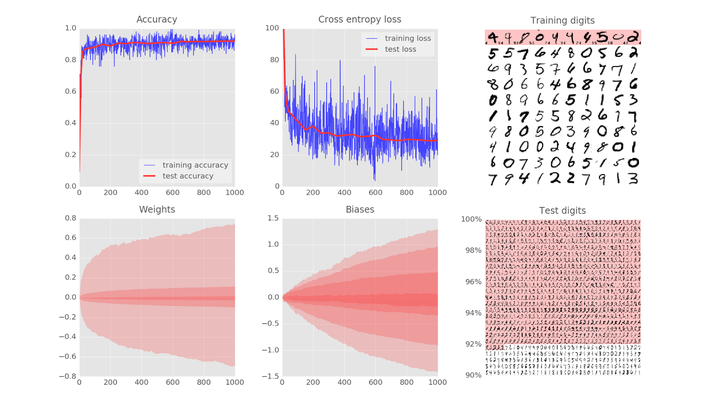3、理论：训练一个神经网络

Training digits => updates to weights and biases => better recognition (loop)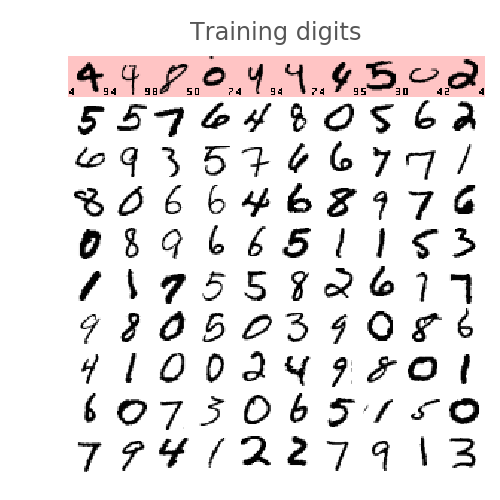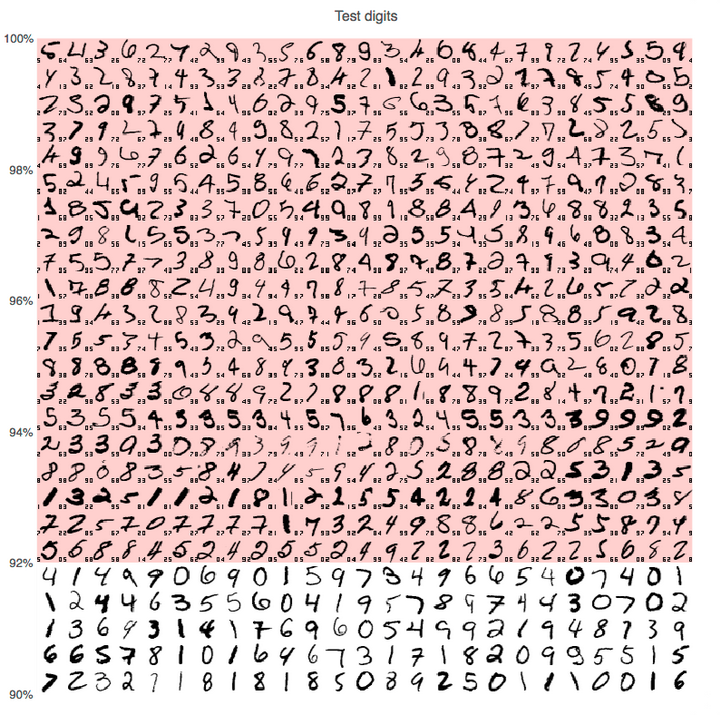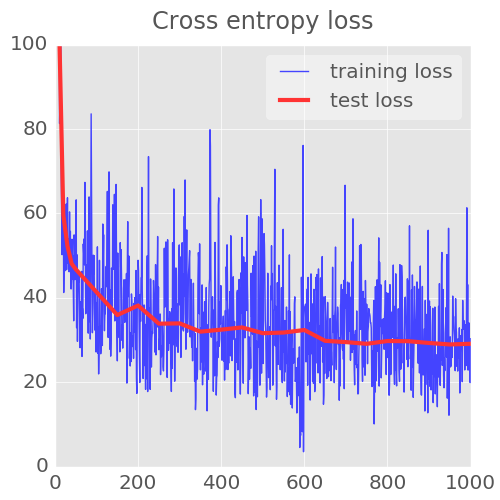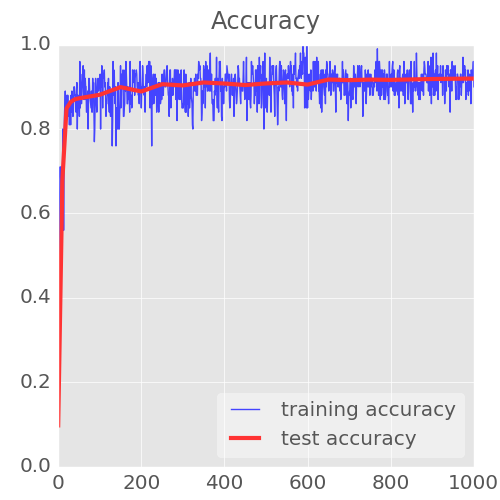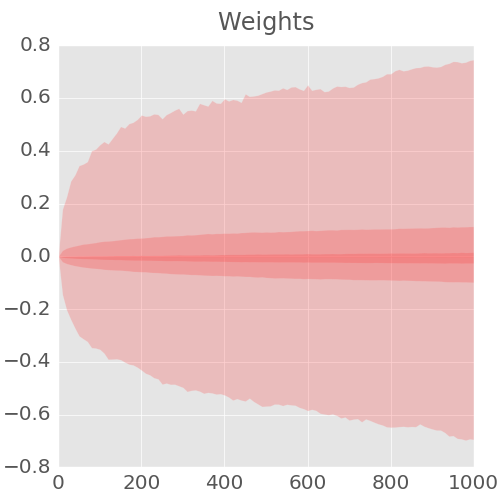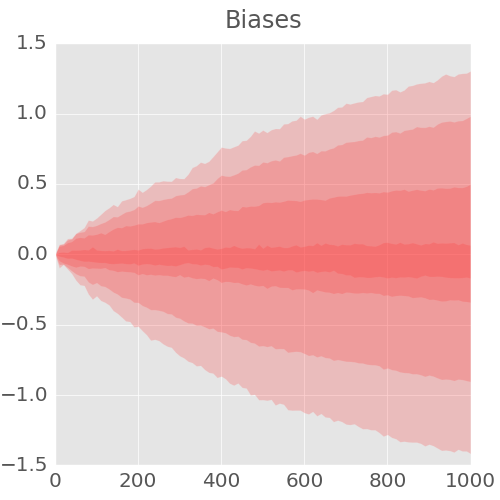1 ......... display 1st graph only 仅显示第 1 张图
2 ......... display 2nd graph only 仅显示第 2 张图
3 ......... display 3rd graph only 仅显示第 3 张图
4 ......... display 4th graph only 仅显示第 4 张图
5 ......... display 5th graph only 仅显示第 5 张图
6 ......... display 6th graph only 仅显示第 6 张图
7 ......... display graphs 1 and 2 显示 1 和 2 图
8 ......... display graphs 4 and 5 显示 4 和 5 图
9 ......... display graphs 3 and 6 显示 3 和 6 图
ESC or 0 .. back to displaying all graphs 返回，显示所有图

O ......... box zoom mode (then use mouse) 框缩放模式（然后使用鼠标）
H ......... reset all zooms 重置所有缩放
Ctrl-S .... save current image 保存当前图像

4、理论 : 单层神经网络MNIST 数据集中，手写数字是 28x28 像素的灰度图像。将它们进行分类的最简单的方法就是使用 28x28=784 个像素作为单层神经网络的输入。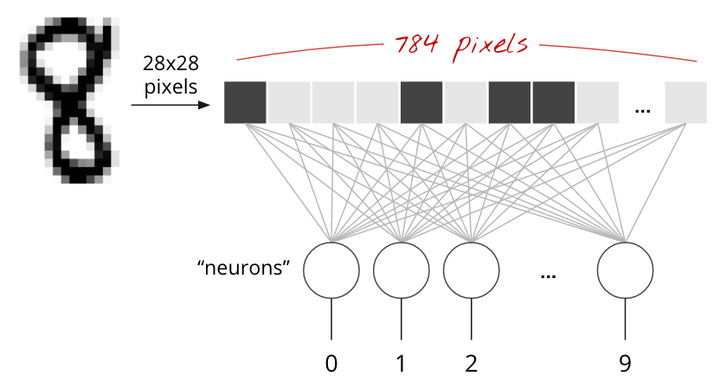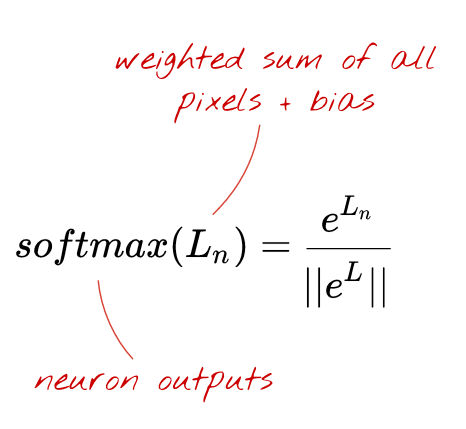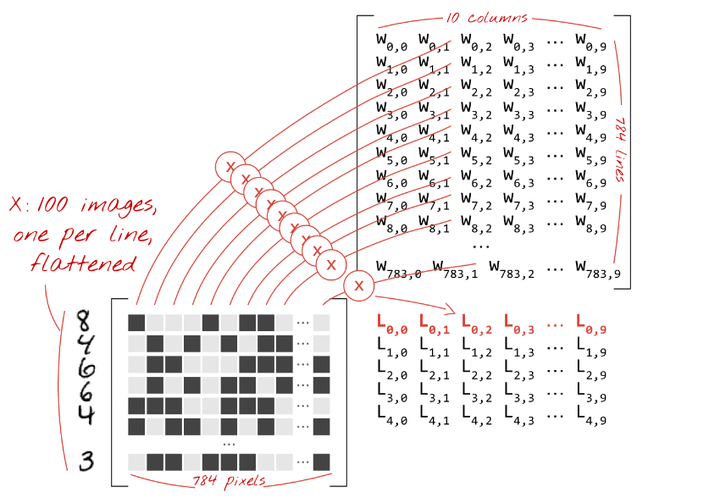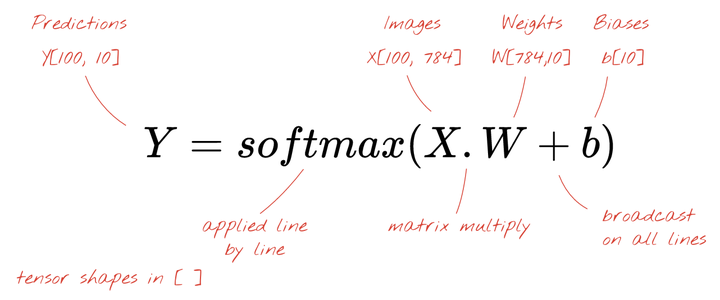「张量（tensor）」像一个矩阵，但是却有着任意数量的维度。一个 1 维的张量是一个向量。一个二维的张量是一个矩阵。然后你可以有 3, 4, 5 或者更多维的张量。

5、理论：梯度下降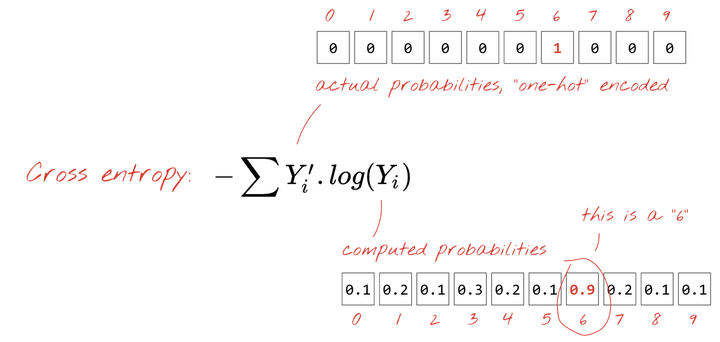「one-hot」编码意味着你使用一个 10 个值的向量，其中除了第 6 个值为 1 以外的所有值都是 0。这非常方便，因为这样的格式和我们神经网络预测输出的格式非常相似，同时它也作为一个 10 值的向量。

「训练」一个神经网络实际上意味着使用训练图像和标签来调整权重和偏置，以便最小化交叉熵损失函数。它是这样工作的。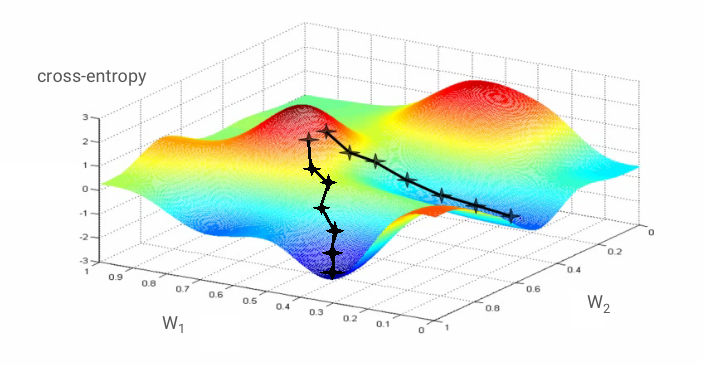「学习率（learning rate）」： 在整个梯度的长度上，你不能在每一次迭代的时候都对权重和偏置进行更新。这就会像是你穿着七里靴却试图到达一个山谷的底部。你会直接从山谷的一边到达另一边。为了到达底部，你需要一些更小的步伐，即只使用梯度的一部分，通常在 1/1000 区域中。我们称这个部分为「学习率（Learning rate）」。

Training digits and labels => loss function => gradient (partial derivatives) => steepest descent => update weights and biases => repeat with next mini-batch of training images and labels

6、实验：让我们来看看代码

mnist_1.0_softmax.py：

import tensorflow as tfX = tf.placeholder(tf.float32, [None, 28, 28, 1])W = tf.Variable(tf.zeros([784, 10]))b = tf.Variable(tf.zeros())init = tf.initialize_all_variables()

• 28, 28, 1: 图像是 28x28 每像素 x 1（灰度）。最后一个数字对于彩色图像是 3 但在这里并非是必须的。
• None: 这是代表图像在小批量（mini-batch）中的数量。在训练时可以得到。

mnist_1.0_softmax.py：

# modelY = tf.nn.softmax(tf.matmul(tf.reshape(X, [-1, 784]), W) + b)# placeholder for correct labelsY_ = tf.placeholder(tf.float32, [None, 10])# loss functioncross_entropy = -tf.reduce_sum(Y_ * tf.log(Y))# % of correct answers found in batchis_correct = tf.equal(tf.argmax(Y,1), tf.argmax(Y_,1))accuracy = tf.reduce_mean(tf.cast(is_correct, tf.float32))

mnist_1.0_softmax.py：

optimizer = tf.train.GradientDescentOptimizer(0.003)train_step = optimizer.minimize(cross_entropy)

TensorFlow 的 “延迟执行（deferred execution）” 模型：TensorFlow 是为分布式计算构建的。它必须知道你要计算的是什么、你的执行图（execution graph），然后才开始发送计算任务到各种计算机。这就是为什么它有一个延迟执行模型，你首先使用 TensorFlow 函数在内存中创造一个计算图，然后启动一个执行 Session 并且使用 Session.run 执行实际计算任务。在此时，图形无法被更改。

mnist_1.0_softmax.py：

sess = tf.Session()sess.run(init)for i in range(1000):    # load batch of images and correct answers    batch_X, batch_Y = mnist.train.next_batch(100)    train_data={X: batch_X, Y_: batch_Y}    # train    sess.run(train_step, feed_dict=train_data)

# success ?a,c = sess.run([accuracy, cross_entropy], feed_dict=train_data)

# success on test data ?test_data={X: mnist.test.images, Y_: mnist.test.labels}a,c = sess.run([accuracy, cross_entropy], feed=test_data)
TensorFlow 和 Numpy 是朋友：在准备计算图时，你只需要操纵 TensorFlow 张量和命令，比如 tf.matmul, tf.reshape 等。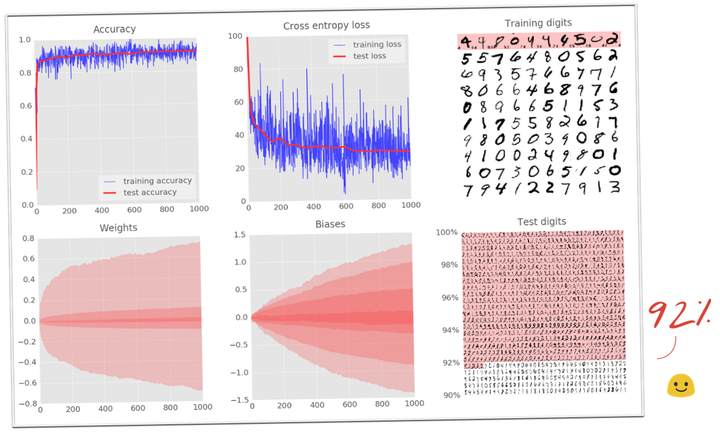7、实验:增加层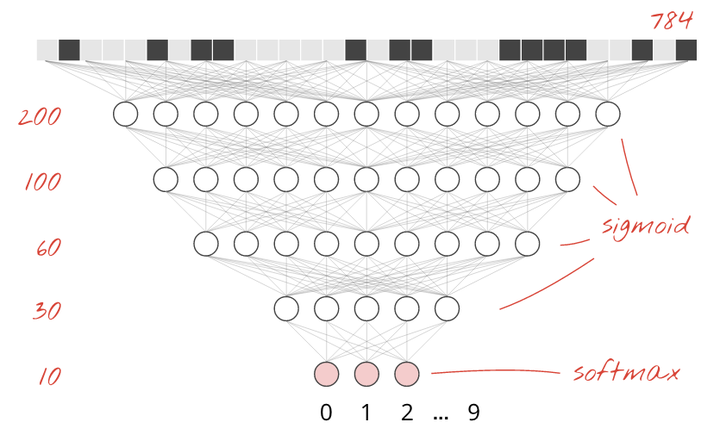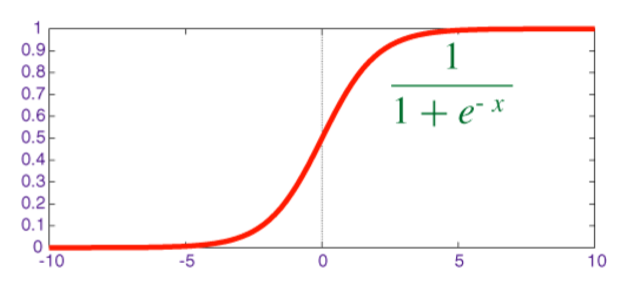W1 = tf.Variable(tf.truncated_normal([28*28, 200] ,stddev=0.1))B1 = tf.Variable(tf.zeros())W2 = tf.Variable(tf.truncated_normal([200, 10], stddev=0.1))B2 = tf.Variable(tf.zeros())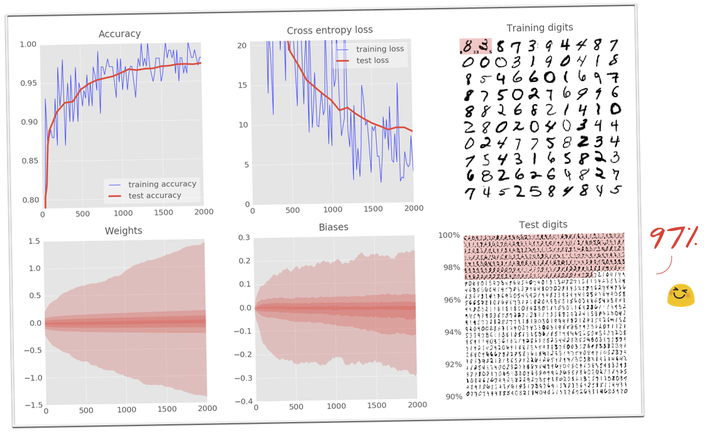8、实验：深度网络需要特别注意的地方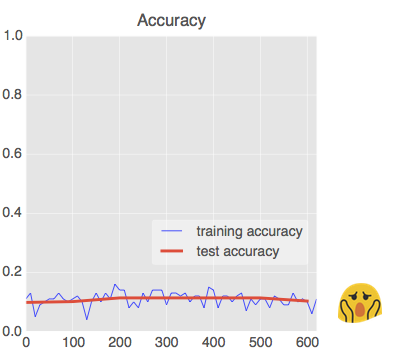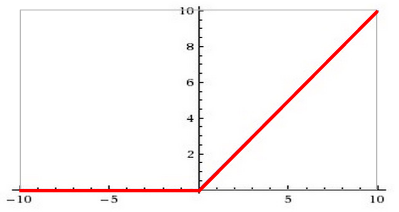W = tf.Variable(tf.truncated_normal([K, L] ,stddev=0.1))B = tf.Variable(tf.ones([L])/10)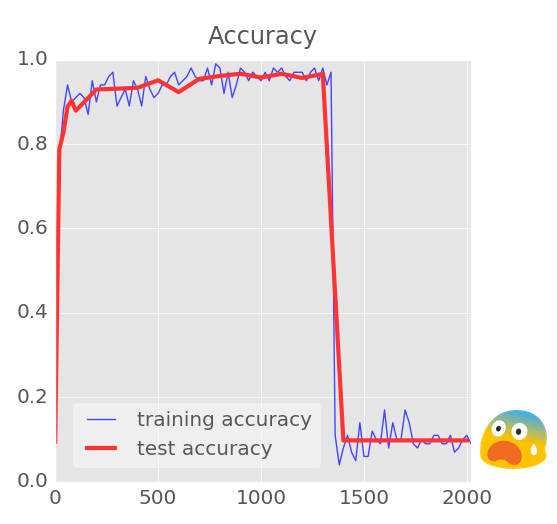Y = tf.nn.softmax(tf.matmul(Y4, W5) + B5)

Ylogits = tf.matmul(Y4, W5) + B5Y = tf.nn.softmax(Ylogits)

cross_entropy = tf.nn.softmax_cross_entropy_with_logits(Ylogits, Y_)

cross_entropy = tf.reduce_mean(cross_entropy)*100

9、实验：学习速率衰退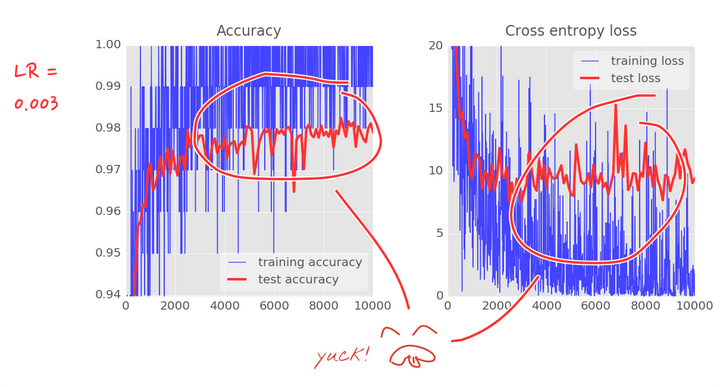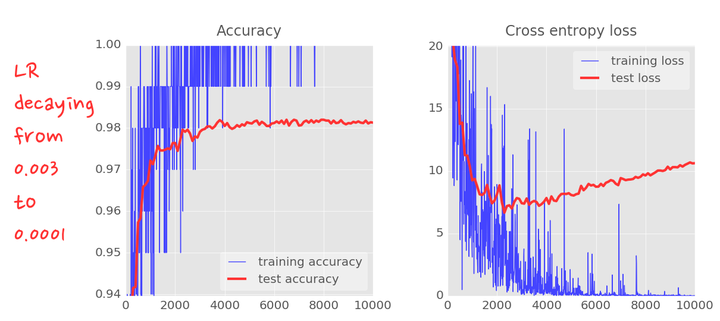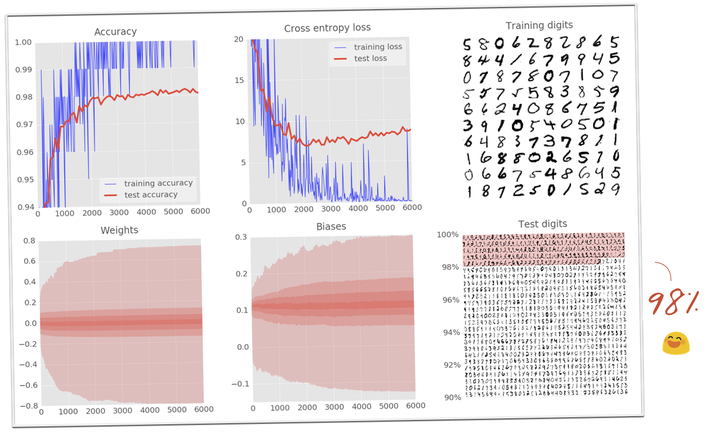10、实验：dropout、过拟合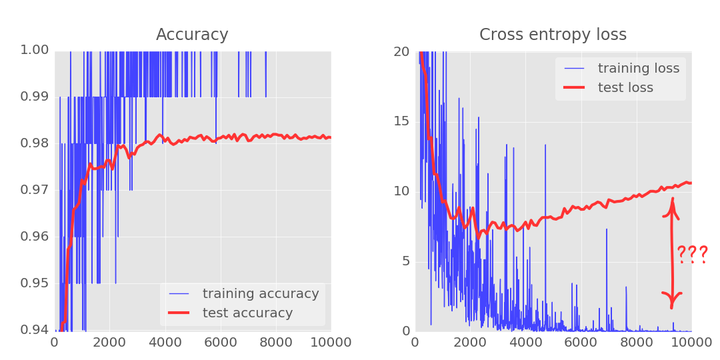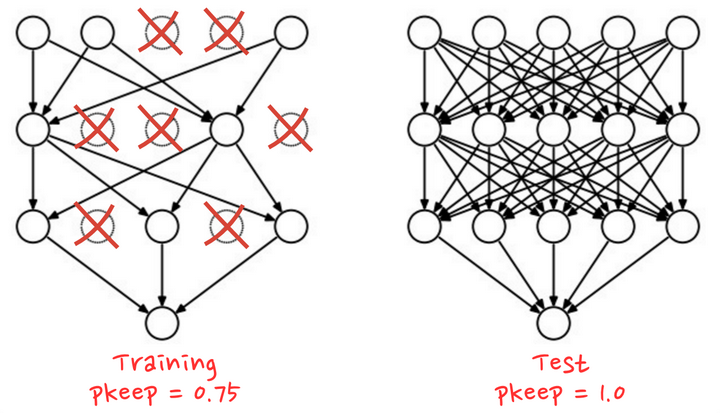TensorFlow 提供一个 dropout 函数可以用在一层神经网络的输出上。它随机地清零一些输出并且把剩下的提升 1/pkeep。这里是如何把它用在一个两层神经网络上的例子。
# feed in 1 when testing, 0.75 when trainingpkeep = tf.placeholder(tf.float32)Y1 = tf.nn.relu(tf.matmul(X, W1) + B1)Y1d = tf.nn.dropout(Y1, pkeep)Y = tf.nn.softmax(tf.matmul(Y1d, W2) + B2)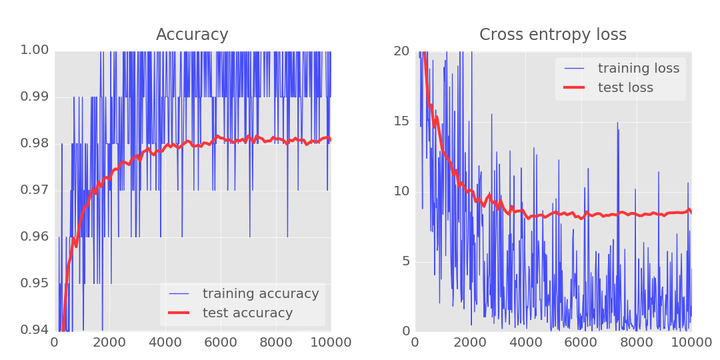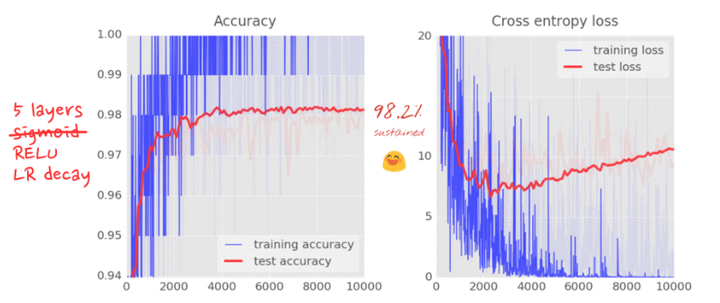11、理论：卷积网络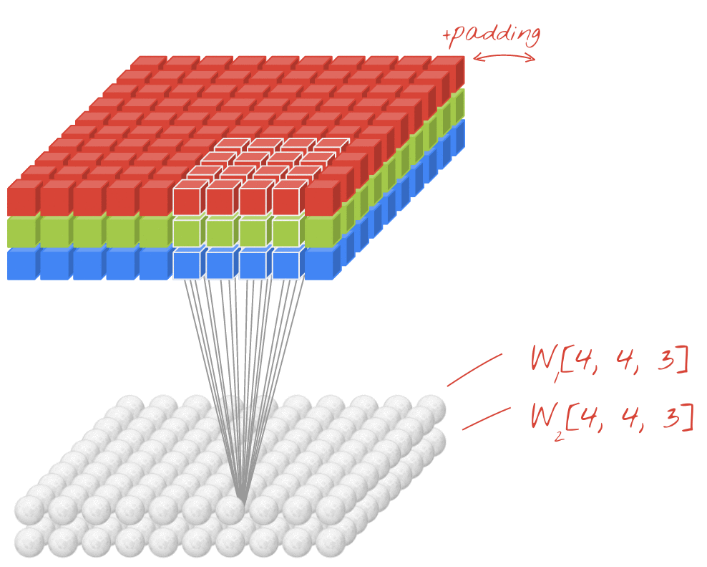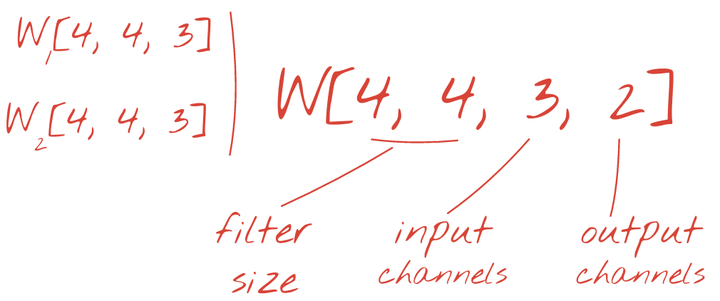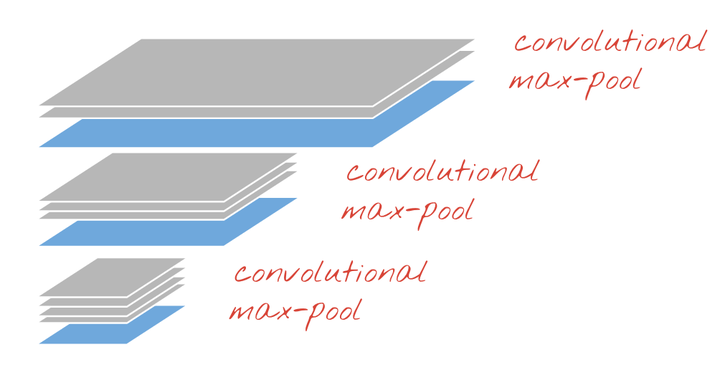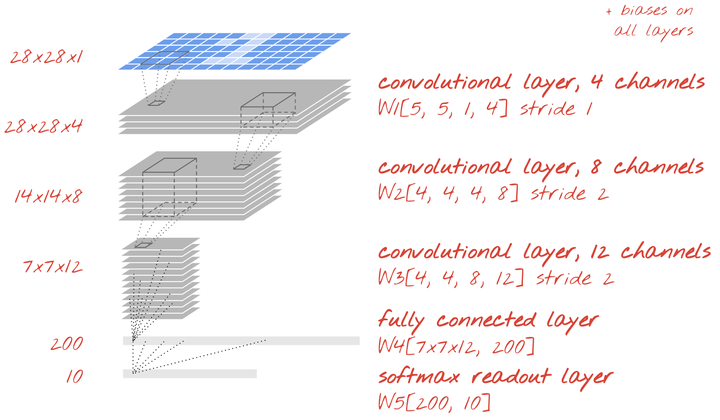12、实现：一个卷积网络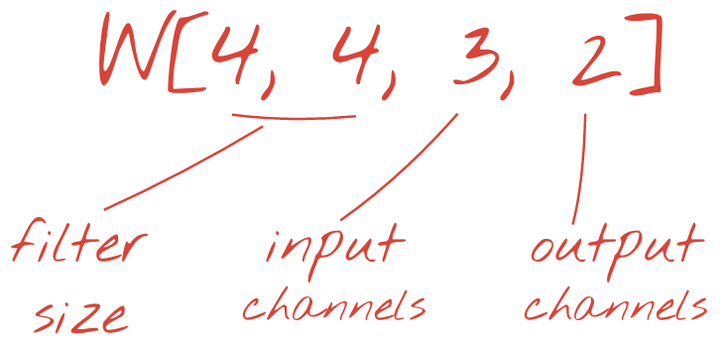W = tf.Variable(tf.truncated_normal([4, 4, 3, 2], stddev=0.1))B = tf.Variable(tf.ones()/10) # 2 is the number of output channels

stride = 1  # output is still 28x28Ycnv = tf.nn.conv2d(X, W, strides=[1, stride, stride, 1], padding='SAME')Y = tf.nn.relu(Ycnv + B)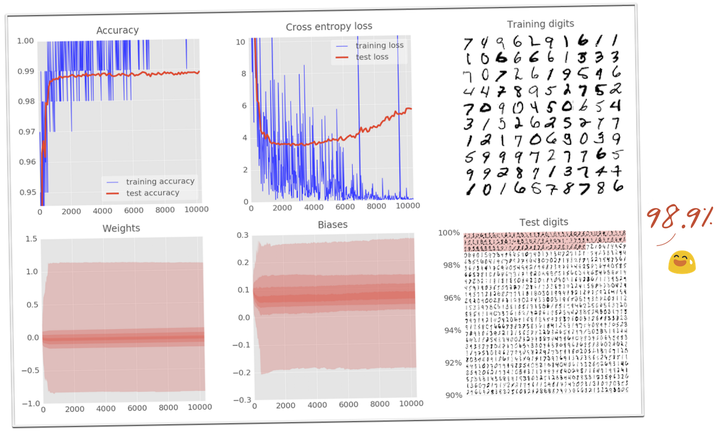13、99% 准确率的挑战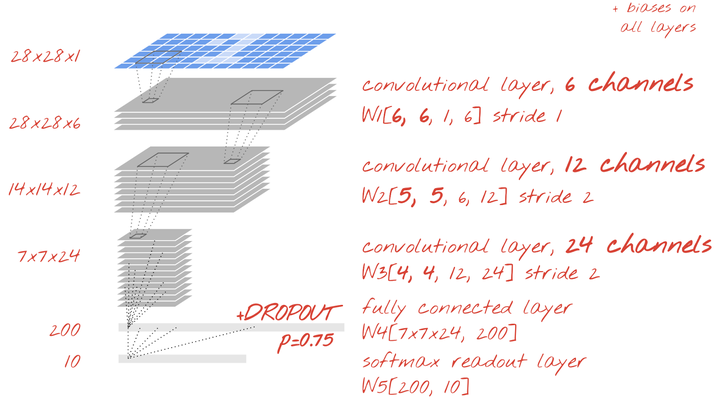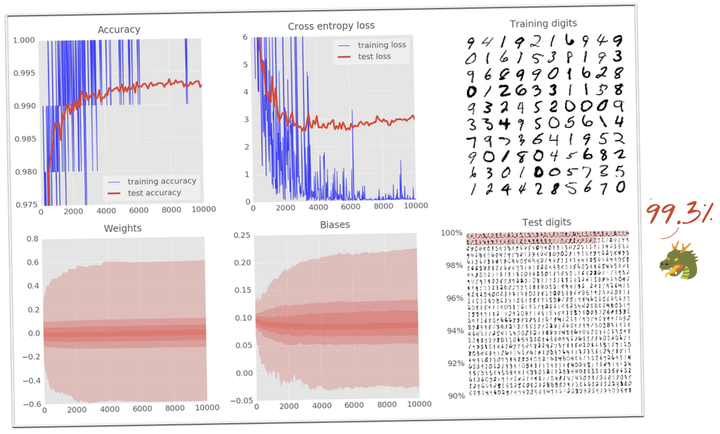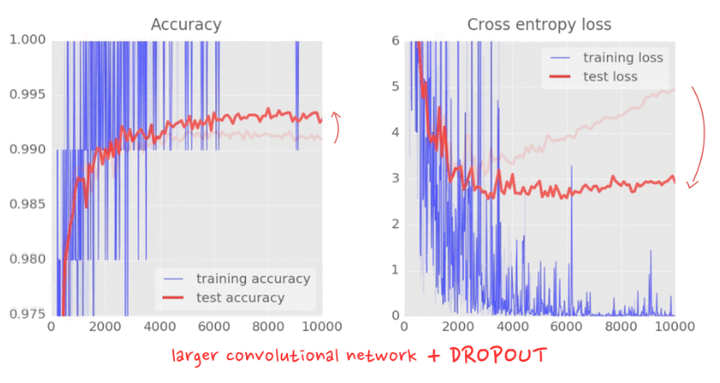14、恭喜！精彩评论9

 代码的格式好怪呀~ 麻烦修改一下哈~ 看着好累~

liangyongming  TF荚荚  发表于 2018-4-14 14:38:33 | 显示全部楼层
 有点乱，但还是谢谢楼主！收获了一点知识点，但由于是小白，真不知道如何搞，如果搞个小白版手把手教学的文章就好了！

chenglu  TF荚荚  发表于 2018-4-14 17:03:54 | 显示全部楼层
 好文章，但是建议非原创性文章不要做全文转载，可以留一个原始链接，大家去那里看。但是可以在这里讨论。M丶Sulayman  TF豆豆  发表于 2018-4-14 21:31:37 | 显示全部楼层
 建议不要直接粘贴复制过来，图片加载都失败了

 统一回复下：①文章非原创，没有注明，这就补上 ②原文链接是有的，第一句蓝色字体就是，点击进去就能看到原文 ③排版问题：编辑框里图片显示正常，发布后不一样了 ④为什么要全文转载？缩小大家的阅读路径

 舟3332 发表于 2018-4-14 08:30 代码的格式好怪呀~ 麻烦修改一下哈~ 看着好累~ 看原文吧（蓝色链接），discuz论文图片加载有点问题

 liangyongming 发表于 2018-4-14 14:38 有点乱，但还是谢谢楼主！收获了一点知识点，但由于是小白，真不知道如何搞，如果搞个小白版手把手教学的文 ... 这篇文章算是中级版，小白建议看我发的另外一篇文章《小白都能懂的tensorflow入门》

M丶Sulayman  TF豆豆  发表于 2018-4-16 08:47:39 | 显示全部楼层
 王奇文 发表于 2018-4-15 18:24 统一回复下：①文章非原创，没有注明，这就补上 ②原文链接是有的，第一句蓝色字体就是，点击进去就能看到 ... 辛苦辛苦~THXneverchange  TF芽芽  发表于 2018-7-4 15:21:11 | 显示全部楼层
 已标记的转载的，应该附带原文链接本版积分规则 回帖后跳转到最后一页